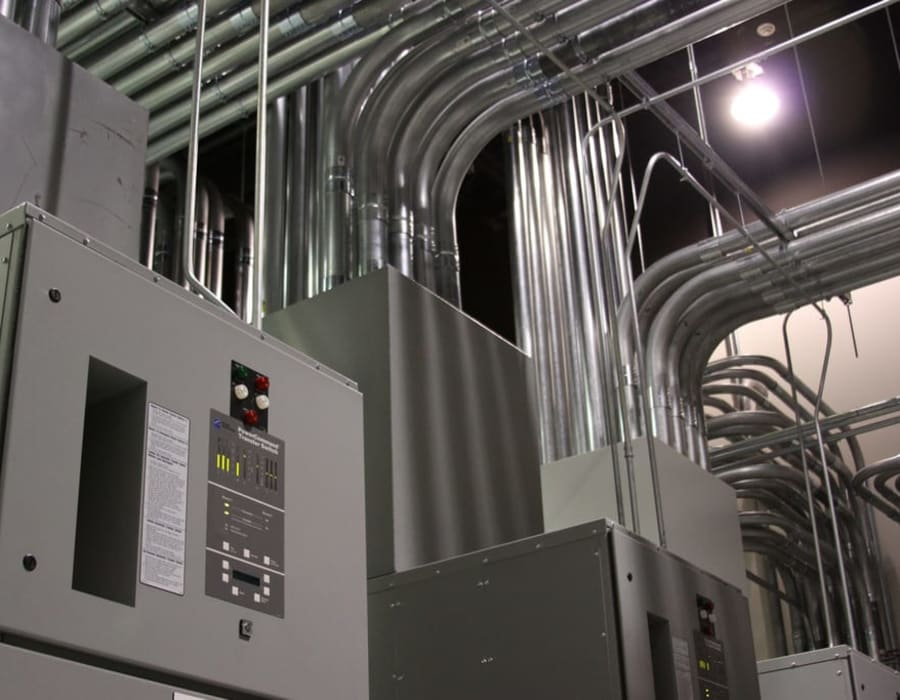# How to Convert and Calculate Power for Crypto Miners… What is a Kilowatt vs Kilowatt Hour and Megawatt?

30 May 2018 by Bob West### Converting Kilowatts (kW) and Watts (W)

A kilowatt (kW) is a standard measurement of power for any electrical device. Most of the time we measure our electronics in watts (W). However, when you go over a certain number of watts it’s easier to use kilowatts. Because a kilowatt is equal to 1,000 watts you can simply say that it is 1 kilowatt or 1 kW. To calculate kW and W simply divide or multiply by 1,000.

2 kW = 2,000 W (2 kW x 1,000 = 2,000 W)

2,000 W = 2 kW (2,000 W / 1,000 = 2 kW)

### Converting kilowatts and Megawatts (MW)

Megawatts, kilowatts and watts are all divisible by 1,000. A megawatt is equal to 1,000 kilowatts and 1,000,000 watts. Here’s how it works. To get the number of megawatts from kilowatts you will want to divide by 1,000 just like in the examples above. To get the number of kilowatts from megawatts you will want to multiply by 1,000.

2 MW = 2,000 kW (2 MW x 1,000 = 2,000 kW)

2,000 kW = 2 MW (2,000 kW / 1,000 = 2 MW)

2 MW = 2,000,000 W (2 MW x 1,000,000 = 2,000,000 W)

2,000,000 W = 2 MW (2,000,000 W / 1,000,000 = 2 MW)

### Calculating kilowatt Hours (kWh)

As you can see, it’s easy to go back and forth from kilowatts to watts. But what about kilowatt hours (kWh)? Kilowatt hours is a little more complex because it involves both power and time. There are several factors to consider but follow these steps to get your kWh.

1. Find the wattage on your mining rig
2. Multiply wattage by hours used each day. (24 hours)
3. Divide by 1,000 (watts to kilowatts)
4. Multiply by number of days (30 days)

Bitmain Antminer S9 Example

1. 1,323 Watts
2. 1,323 Watts x 24 Hours = 31,752
3. 31,752 / 1,000 = 31.752 kWh per day
4. 31.752 kWh per day x 30 days = 952.56 kWh per month

### Monthly Cost of Electricity (kWh x Per/ kWh)

To calculate this, you will have to get your cost per kWh from the utility company or from your last statement.It can be hard to figure out the actual cost per kWh because of tiers, fees, taxes and so forth. It may be helpful to contact your utility company to get a better idea of the cost per kWh.

Example: .0678 cents per kWh

1. 952.56 kWh per month
2. 952.56 kWh per month x .0678 cents per kWh = \$64.58

It will cost you approximately 64.58 cents per Bitmain Antminer S9 in electricity at a cost of .0678 cents per kWh.

### Monthly Price Per kW to Price Per Mining Rig

Another common question is what does the kilowatt cost per month equal per mining rig? To calculate this, you will simply get the cost per kW from the datacenter or mining farm. Typically, prices range from \$80-\$140 per kW per month. This includes the space, power, internet, cooling and security.

You will want to get your cost per kW per month. Next, you will want to calculate your total power demand. This should include your load factor of 80% if you are in the United States. This is a requirement among most data centers and mining farms anyway. For safety, it should also apply to at home use and commercial buildings.

1. Determine total power demand in watts
2. Multiply total power demand in watts x load factor (80% or 1.2)
3. Convert watts (W) to kilowatts (kW)
4. Multiply your total power demand including load factor x price per kW / month

Example: \$85 per kW / Month, 80% Load Factor, 100 Bitmain Antminer S9s

1. 100 x 1,323 Watts = 132,300 Watts
2. 132,300 Watts x 1.2 = 158,760 Watts
3. 158,760 Watts / 1,000 = 158.76 Kilowatts (kW)
4. 158.76 kW x \$85 = \$13,494.60 per month

In the example above, it will cost you \$13,494 per month to host 100 Bitmain Antminer S9s at \$85 per kW with power requirement of 1,323 watts per mining rig. This includes the load factor which adds an additional 20% power demand to your power requirements.

It’s important to note that the reason for the load factor is that the breakers and wires cannot take a continuous load. It’s like redlining the engine of your car for too long.

Don't Forget Cooling and Other Costs!

The above calculations are for the electricity costs of mining rigs only and do not take into consideration the cost to run and cool the mining farm for data center facility. GPU and especially ASIC mining rigs put out a lot of heat - a lot more than servers and traditional equipment found in enterprise data centers.

I will be co-authoring another article on the costs associated with running and cooling a data center facility and how that impacts the cost per kWh with another LinkedIn friend. Please stay tuned on that article and make sure to share your feedback and comments below.

Keyword Tags: cryptocurrency, cryptocurrency mining, bitcoin mining, bitcoin mining hosting, asic miner hosting, gpu miner hosting, bitcoin colocation, wholesale colocation, data center, data centers, mining farms, bitcoin mining farms, crypto, crypto mining farms

Author

Bob West

Bob West a technology savvy digital marketing executive and entrepreneur specializing in the latest data center and cloud technologies for business. His background includes the initial development of Datacenters.com in 2011. He’s a cryptocurrency mining expert, data center, and cloud enthusiast.